# Octahedron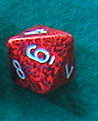An octahedron is a solid shape that looks like two square pyramids glued together on their bases. The crystals of some minerals, such as magnetite, can be shaped like octahedra (the plural of octahedron). There's a picture of some magnetite crystals on the right.

If you've ever played role playing games or know someone who has, then you might have seen a die shaped like an octahedron (see the one on the left).

So, what is a icosahedron, and what are its properties?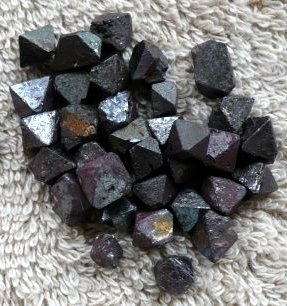## The Properties of an Octahedron

An octahedron is a polyhedron with 8 flat faces. Its name comes from the Greek prefix octa, which means 8.

It has 8 faces, 12 edges and 6 vertices (corner points). In a regular octahedron, 4 edges meet at each vertex.

Each face of a regular octahedron is an equilateral triangle, so it has 3 edges.

A regular octahedron is one of the $5$ Platonic solids.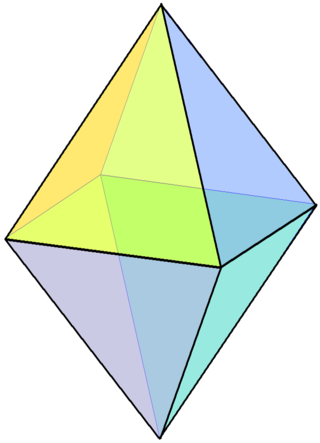## Building an Octahedron

You can make a octahedron yourself by using a template like the following net (template), cutting it out along the outside lines, folding it along the inside lines and gluing it together on the tabs.## Finding the Surface Area and Volume of a Regular Octahedron

Because a regular octahedron is a regular polyhedron, every edge of the octahedron has the same length.

### Finding the Surface Area of a Icosahedron

The regular octahedron has $8$ faces, each of which is shaped like an equilateral triangle. As the area of an equilateral triangle is equal to $\dfrac{\sqrt{3}}{4}(\text{edge-length})^2$, the surface area of a regular icosahedron is

$\text{Surface Area} = 8 \times \left(\dfrac{\sqrt{3}}{4}(\text{edge-length})^2\right) = 2 \sqrt{3} (\text{Edge-Length})^2.$

For example, the surface area of an octahedron with edge length $4$ cm is

\begin{align*} \text{Surface Area} &=2 \sqrt{3}(\text{Edge-Length})^2\\ &= 2 \sqrt{3} (4)^2\\ &= 32 \sqrt{3} \\ &\approx 55.4 \text{ cm}^2 \end{align*}
You probably don't need to memorise this formula. Being able to look it up is more than enough.

### Finding the Volume of a Regular Octahedron

The volume of a regular octahedron is given by the formula

$\text{Volume} = \dfrac{\sqrt{2}}{3} (\text{Edge-Length})^3$

For example, the volume of an octahedron with edge-length $3$ cm is

\begin{align*} \text{Volume} &= \dfrac{\sqrt{2}}{3}(\text{Edge-Length})^3\\ &= \dfrac{\sqrt{2}}{3}(3)^3\\ &= 9 \sqrt{2} \\ &\approx 12.73 \text{ cm}^3 \end{align*}
Similarly, just know where to find the volume formula. Don't bother trying to memorise it.

### Irregular Octahedra

Although we've really only talked about regular octahedra in this article, octahedra can also be irregular like the one in the picture below: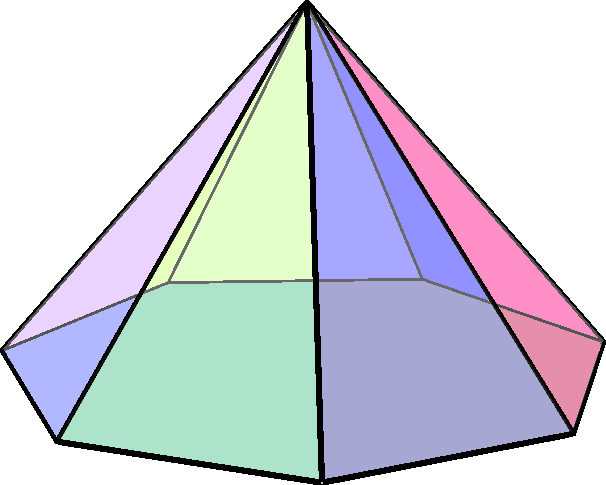Do you notice the faces with different shapes? This solid has another name. It is a heptagonal pyramid as its base has seven sides. It's probably more descriptive to call it a heptagonal pyramid, but it is an octahedron, nonetheless. A hexagonal prism is also an irregular octahedron. A squashed regular octahedron would also be irregular.

### Dual of an Octahedron (Some Advanced Geometry)

Mathematicians are interested in finding the duals of polyhedra. The dual of an octahedron is found by taking the midpoints of its faces as the vertices of another polyhedron. If you join up the midpoints of the faces of an octahedron, you get a cube. If you join up the midpoints of the faces of a cube, you get an octahedron. The cube and octahedron are dual polyhedra.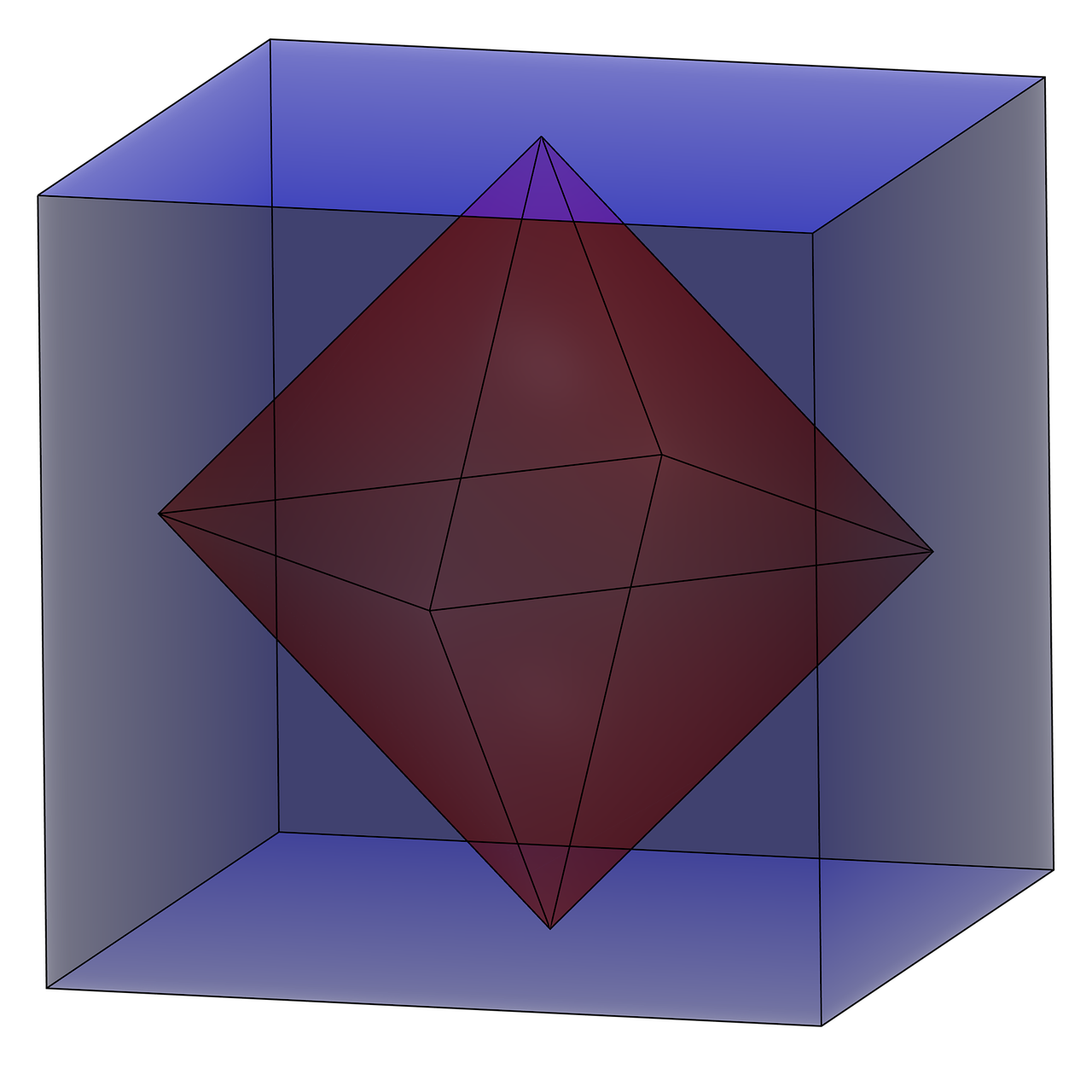### Description

There are several lessons related to 3D geometry such as

1. Euler's formula
2. Vertices, Edges and Faces
3. Volumes of 3D shapes
4. etc

Even though we've titled this lesson series to be more inclined for Year 10 or higher students, however, these lessons can be read and utilized by lower grades students.

### Prerequisites

Understanding of 3D shapes

### Audience

Year 10 or higher, but suitable for Year 8+ students

### Learning Objectives

Get to know 3D Geometry

Author: Subject Coach
You must be logged in as Student to ask a Question.